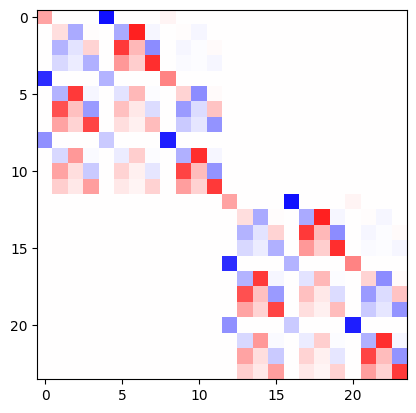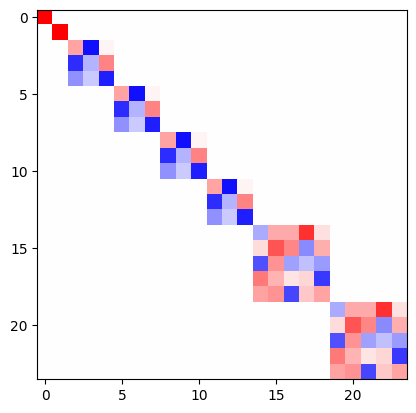# Euclidean neural networks

## What is e3nn?

e3nn is a python library based on pytorch to create equivariant neural networks for the group $$O(3)$$.

## Demonstration

All the functions to manipulate rotations (rotation matrices, Euler angles, quaternions, convertions, …) can be found here Parametrization of Rotations. The irreducible representations of $$O(3)$$ (more info at Irreps) are represented by the class e3nn.o3.Irrep. The direct sum of multiple irrep is described by an object e3nn.o3.Irreps.

If two tensors $$x$$ and $$y$$ transforms as $$D_x = 2 \times 1_o$$ (two vectors) and $$D_y = 0_e + 1_e$$ (a scalar and a pseudovector) respectively, where the indices $$e$$ and $$o$$ stand for even and odd – the representation of parity,

import torch
from e3nn import o3

irreps_x = o3.Irreps('2x1o')
irreps_y = o3.Irreps('0e + 1e')

x = irreps_x.randn(-1)
y = irreps_y.randn(-1)

irreps_x.dim, irreps_y.dim

(6, 4)


their outer product is a $$6 \times 4$$ matrix of two indices $$A_{ij} = x_i y_j$$.

A = torch.einsum('i,j', x, y)
A

tensor([[ 0.9392, -1.5069, -0.8065, -0.2333],
[ 1.2374, -1.9854, -1.0626, -0.3074],
[-0.3351,  0.5376,  0.2877,  0.0832],
[ 0.1121, -0.1799, -0.0963, -0.0278],
[-1.6751,  2.6876,  1.4384,  0.4161],
[-0.6226,  0.9990,  0.5347,  0.1547]])


If a rotation is applied to the system, this matrix will transform with the representation $$D_x \otimes D_y$$ (the tensor product representation).

$A = x y^t \longrightarrow A' = D_x A D_y^t$

Which can be represented by

R = o3.rand_matrix()
D_x = irreps_x.D_from_matrix(R)
D_y = irreps_y.D_from_matrix(R)

plt.imshow(torch.kron(D_x, D_y), cmap='bwr', vmin=-1, vmax=1);This representation is not irreducible (is reducible). It can be decomposed into irreps by a change of basis. The outerproduct followed by the change of basis is done by the class e3nn.o3.FullTensorProduct.

tp = o3.FullTensorProduct(irreps_x, irreps_y)
print(tp)

tp(x, y)

FullTensorProduct(2x1o x 1x0e+1x1e -> 2x0o+4x1o+2x2o | 8 paths | 0 weights)

tensor([-1.4355,  0.8159,  0.9392,  1.2374, -0.3351,  0.1121, -1.6751, -0.6226,
-0.4208,  0.5451,  0.8336, -0.0839,  0.7261, -1.9685,  0.2152, -1.9742,
-0.2864, -0.0139,  1.1244,  0.6867,  1.8324,  1.1848,  0.6723,  0.2366])


As a sanity check, we can verify that the representation of the tensor prodcut is block diagonal and of the same dimension.

D = tp.irreps_out.D_from_matrix(R)
plt.imshow(D, cmap='bwr', vmin=-1, vmax=1);e3nn.o3.FullTensorProduct is a special case of e3nn.o3.TensorProduct, other ones like e3nn.o3.FullyConnectedTensorProduct can contained weights what can be learned, very useful to create neural networks.